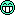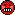## Numerical Deconvolution [Dissolution / BCS / IVIVC]

Dear Daniel!

❝ So, if possible, I would like to know how apply deconvolution approach to obtain fraction absorbed using excel. This method is known as model-independent approach. Could you help me with details about calculations and articles about this topic?

First I would recommend two other ‘classical’ papers:
1. Vaughan DP, Dennis M. Mathematical Basis of Point-Area Deconvolution Method for Determining In Vivo Input Functions. J Pharm Sci. 1978; 67: 663–5.
2. Langenbucher F. Numerical Convolution/Deconvolution as a Tool for Correlating in Vitro with in Vivo Drug Availability. Pharm Ind. 1982; 44(11): 1166–72.
Deconvolution is not necessarily model-independent; there are two versions:
1. A model-dependent version, where models are defined for the functions, and the convolution integral solved for the unknown function by means of Laplace Transformations. Unfortunatelly the integral may be impossible to solve…
2. A model-independent version, where the data are deconvoluted numerically, i.e. differentials are replaced by differences.
You also might get an impression by an M$-Excel-sheet I prepared, which uses an example from Langenbucher (in German and out of print)…Langenbucher F. In vitro Prüfung und Korrelation mit in vivo Daten. In: Meier J, Rettig H, Hess H. Biopharmazie – Theorie und Praxis der Pharmakokinetik. Stuttgart 1981; Georg Thieme: pp 300–3. Main problems with numerical deconvolution are: Sampling points of the response function (e.g., an oral formulation), and the weighting function (i.e., an i.v. dose or an oral solution) must be equidistant, which they almost never are. Therefore you must interpolate ‘missing’ data points by a suitable algorithm (first approximation would be linear interpolation until Tmax, and linear/log afterwards, better options are splines or Lagrange-polynoms). Numerical deconvolution is subject to instability such as sudden oscillations in the input function. Errors in early time points have a cumulative effect upon subsequent estimates. You may use my speadsheet as some kind of a sandbox: alter values, and watch…But please don’t use it for your actual problems! For the application of M$-Excel in IVIVC have a look at

Langenbucher F. Handling of computational in vitro/in vivo correlation problems by Microsoft Excel: III. Convolution and deconvolution. Eur J Pharm Biopharm. 2003; 56(3): 429–37. doi:10.1016/s0939-6411(03)00140-1.

Software packages having a deconvolution module onboard (WinNonlin 5.2+, Kinetica, PDx-IVIVC) rely heavily on smoothing of data – but manufacturers rarely document the actual method in the manual.Good look!

Dif-tor heh smusma 🖖🏼 Довге життя Україна!Helmut SchützThe quality of responses received is directly proportional to the quality of the question asked. 🚮
Science QuotesIng. Helmut Schütz